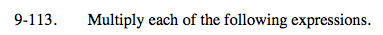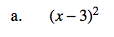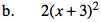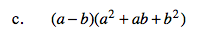Home > A2C > Chapter 9 > Lesson 9.2.3 > Problem9-113

9-113.
1. Multiply each of the following expressions. Homework Help ✎

1. (x − 3)2

2. 2(x + 3)2

3. (a − b)(a2 + ab + b2)x 2− 6x + 92x 2 + 12x + 18a3b3